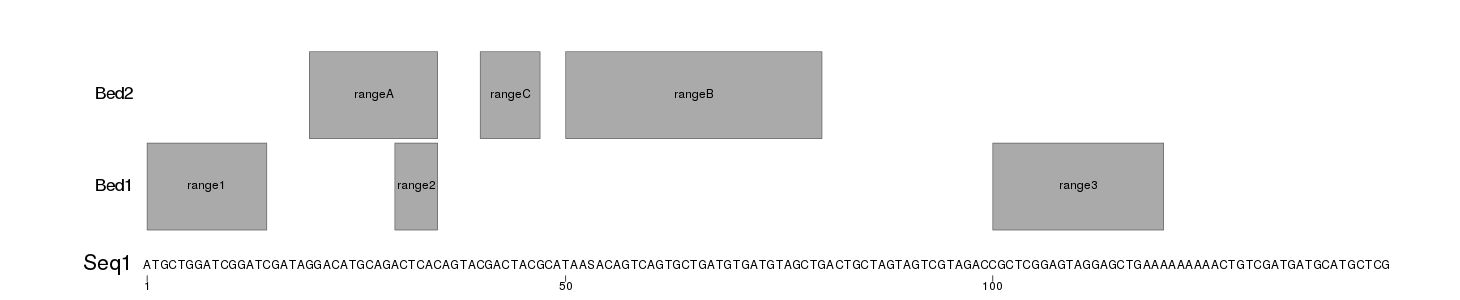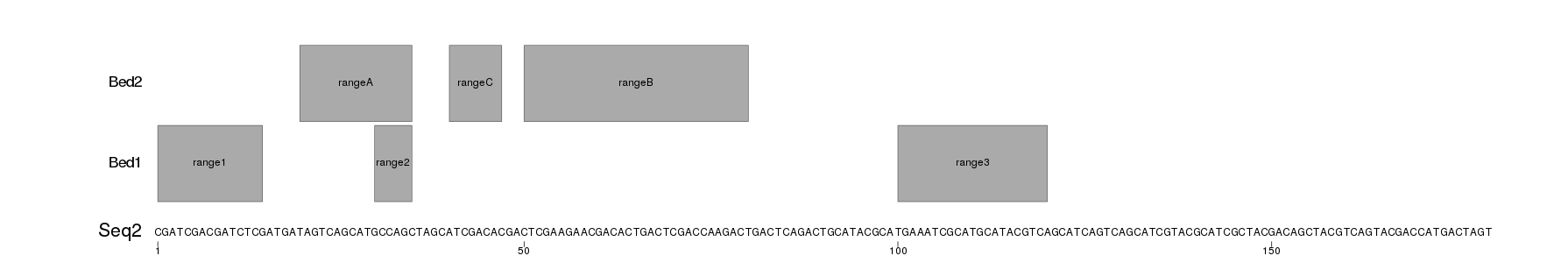Question: Visualize short nucleotide sequences and some ranges on top
1
mschmid150 wrote:

I have a FASTA file with lots of short nucleotide sequences up to about 1000bp. In addition I have several BED files corresponding to those sequences.

Now I want to plot the sequences as the base track with the BED files as tracks on top. I want the actual sequence to be visualized ("ATGCTTTGCCCCT...", not just a line). And on top I would like to show the ranges given as BED files. Ideally the tool will keep upper/lower case in the FASTA file.

I had a look at Gviz but it seems to be very much focused on the human genome as a reference.

A plus would be something in Python, but R is fine as well.

The layout would be:

``````TRACK N:     <==============>
TRACK 2:              <==============>
TRACK 1:                    <====>
TRACK 0:     ATATATGGGGCTAGCTACGTACGGG....
|        |        |
Pos:         1        10       20
``````

Ideally I can also colour the BED tracks individually and some nucleotides as well.

EDIT: Stressing the fact that I want to plot this to a pdf or other image and NOT for visual inspection. And it has to be automated. Hence it has to be run out of a script.

visualization gff bed • 307 views
modified 11 months ago by bernatgel2.6k • written 11 months ago by mschmid150

Not python or R but IGV may be able do this.

Can it export the individual contigs to a PDF? -- EDIT: And I have to automate this. I can not do this manually :(

Not PDF but you can export images out of IGV programmatically: Automatic IGV snapshot script
One more option here.

2
bernatgel2.6k wrote:

Hi @mschmid,

You can create plots like the ones you ask with [karyoploteR]. It's not trivial, but it's doable.

The key is to define a custom genome with a single chromosome "Cust" going from 1 to the length of the sequence, and then plot the sequence and the bed files on it.

I have created an example with a fasta file (seqs.fa) with two sequences and two ranges files (Bed1.txt and Bed2.txt) with 3 ranges each that will be plotted on top of each sequence. The ranges files are txt and no bed to include the range name in the fourth column.

The following code reads the sequences from the fasta file with Biostrings::readDNAStringSet and the ranges with regioneR::toGRanges. It creates a png file for each sequence adjusting the the png size to the length of the file to the sequence text is readable. To plot, it will defines a custom genome with toGRanges creates the plot with plotKaryotype and then uses kpText and kpRect to plot the sequence and the ranges.

``````library(karyoploteR)
library(Biostrings)

regs <- list(Bed1=toGRanges("Bed1.txt"), Bed2=toGRanges("Bed2.txt"))

#Define the plotting parameters (margins, etc...)
pp <- getDefaultPlotParams(1)
pp\$ideogramheight <- 0
pp\$data1inmargin <- 0
pp\$bottommargin <- 20
pp\$topmargin <- 20

for(i in seq_along(seqs)) {
s <- seqs[i]
seq.name <- names(s)
seq.len <- width(s)
seq.chars <- strsplit(x=as.character(s), "")[]

pngpaste0seq.name, ".png"), width=10*seq.len, height=300)
#Plot with a custom genome
custom.genome <- toGRanges("Cust", 1, seq.len)
kp <- plotKaryotype(genome=custom.genome, ideogram.plotter = NULL, labels.plotter = NULL, plot.params = pp)
kpAddBaseNumbers(kp, tick.dist = 50, minor.ticks = FALSE, cex = 1)

kpAddLabels(kp, labels = seq.name, r0 = 0, r1=0.1, cex=1.8)
for(j in seq_len(seq.len)) {
kpText(kp, chr="Cust", x = j, y=0.05, labels = seq.chars[j])
}

for(j in seq_along(regs)) {
at <- autotrack(j, length(regs), r0=0.2)
kpAddLabels(kp, labels = names(regs)[j], r0=at, cex=1.4)
kpRect(kp, data=regs[[j]], y0=0, y1=1, r0=at, col="#AAAAAA", border="#666666")
kpPlotNames(kp, data=regs[[j]], labels = mcols(regs[[j]])[,1], position = "center", y0=0, y1=1, r0=at)
}

dev.off()
}
``````

This will produce images like these

Seq1.pngSeq2.pngYou would need to customize the appearance, and the data loading, but you should be able to build upon this. You can find more information in the karyoploteR tutorial.

1

THIS. IS. AWESOME. Thanks!

If an answer was helpful, you should upvote it; if the answer resolved your question, you should mark it as accepted. You can accept more than one if they work.